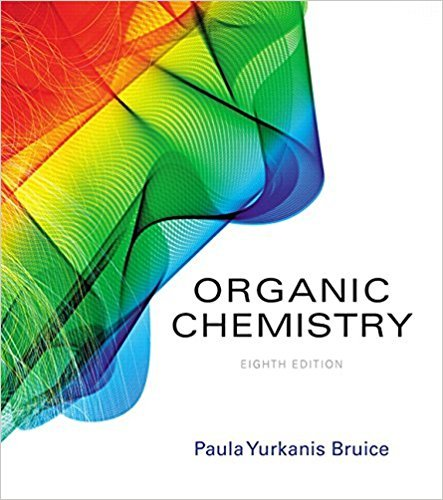×
×

# Solutions for Chapter 15: Reactions of Carboxylic Acids and Carboxylic Acid Derivatives## Full solutions for Organic Chemistry | 8th Edition

ISBN: 9780134042282Solutions for Chapter 15: Reactions of Carboxylic Acids and Carboxylic Acid Derivatives

Solutions for Chapter 15
4 5 0 343 Reviews
12
3
##### ISBN: 9780134042282

Organic Chemistry was written by and is associated to the ISBN: 9780134042282. Chapter 15: Reactions of Carboxylic Acids and Carboxylic Acid Derivatives includes 92 full step-by-step solutions. Since 92 problems in chapter 15: Reactions of Carboxylic Acids and Carboxylic Acid Derivatives have been answered, more than 32881 students have viewed full step-by-step solutions from this chapter. This expansive textbook survival guide covers the following chapters and their solutions. This textbook survival guide was created for the textbook: Organic Chemistry, edition: 8.

Key Chemistry Terms and definitions covered in this textbook
• amphiprotic

Refers to the capacity of a substance to either add or lose a proton 1H+2. (Section 16.2)

• beta elimination

An elimination reaction in which a proton from the beta (b) position is removed together with the leaving group, forming a double bond.

• diol

A compound containing two hydroxyl groups (OH).

• electrostatic potential maps

A three-dimensional, rainbowlike image used to visualize partial charges in a compound.

• Ester

A derivative of a carboxylic acid in which H of the carboxyl group is replaced by a carbon.

• formula weight

The mass of the collection of atoms represented by a chemical formula. For example, the formula weight of NO2 (46.0 amu) is the sum of the masses of one nitrogen atom and two oxygen atoms. (Section 3.3)

• ground state

The lowest-energy, or most stable, state. (Section 6.3)

• heat of reaction

The heat given off during a reaction.

• Hydroperoxide

A compound containing an !OOH group.

• imidazole

A compound containing a five-membered ring that is similar to pyrrole but has one extra nitrogen atom at the 3 position.

• Imine

A compound containing a carbon-nitrogen double bond, R2C"NR’; also called a Schiff base

• Infrared (IR) spectroscopy

A spectroscopic technique in which a compound is irradiated with infrared radiation, absorption of which causes covalent bonds to change from a lower vibration state to a higher one. Infrared spectroscopy is particularly valuable for determining the kinds of functional groups present in a molecule.

• molality

The concentration of a solution expressed as moles of solute per kilogram of solvent; abbreviated m. (Section 13.4)

• nucleotide

Compounds formed from a molecule of phosphoric acid, a sugar molecule, and an organic nitrogen base. Nucleotides form linear polymers called DNA and RNA, which are involved in protein synthesis and cell reproduction. (Section 24.10)

• positron

A particle with the same mass as an electron but with a positive charge, 0 +1e, or b+. (Section 21.1)

• second order

A reaction that has a rate equation in which the sum of all exponents is two.

• secondary cell

A voltaic cell that can be recharged. (Section 20.7)

• spontaneous process

A process that is capable of proceeding in a given direction, as written or described, without needing to be driven by an outside source of energy. A process may be spontaneous even though it is very slow. (Section 19.1)

• Thermodynamic control

Experimental conditions that permit the establishment of equilibrium between two or more products of a reaction. The composition of the product mixture is determined by the relative stabilities of the products.